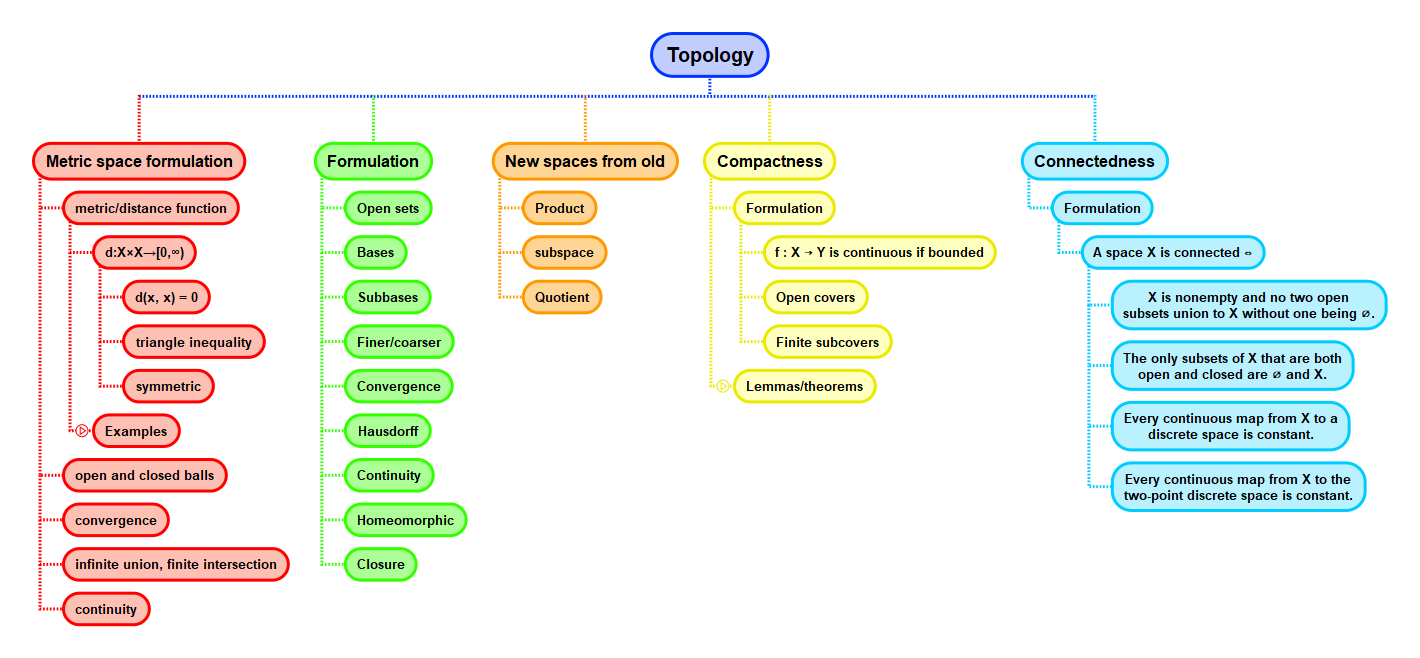Show Question
Math and science::Topology

# Topology and topological space. Definition

A topology on a set $$X$$ is a collection $$\mathcal{T}$$ of subsets of $$X$$ with the following properties:

T1
Whenever $$(U_i)_{i \in I}$$ is a family (finite or not) of subsets of $$X$$ such that $$U_i \in \mathcal{T}$$ for all $$i \in I$$, then  $$\bigcup_{i \in I} U_i \in \mathcal{T}$$.
T2
Whenever $$U_1, U_2 \in \mathcal{T}$$, then $$U_1 \cap U_2 \in \mathcal{T}$$.
T3
$$\emptyset \in \mathcal{T}$$ and $$X \in \mathcal{T}$$.

A topological space $$(X, \mathcal{T})$$ is a pair consisting of a set $$X$$ together with a topology $$\mathcal{T}$$ on $$X$$.

T3 can be derived from T1 and T2, and is not strictly necessary as an axiom; however, most treatments of the subject introduce the definition as this triplet of statements.

### In relaxed words

T1 can be phrased as 'an arbitrary union of open subsets is open'. By induction T2 can be phrased as 'a finite intersection of open subsets is open'.

Combining these two then, a topology on $$X$$ can be defined as 'a collection of subsets of $$X$$ that is closed under arbitrary unions and finite intersections'.

### Discrete and indiscrete topologies

The discrete and indiscrete topologies are two important topologies (which can be defined for any set). With $$X$$ being a set, then they are defined like so:

• the collection of all subsets of $$X$$ is a topology on $$X$$; it is called the discrete topology.
• the collection of subsets of $$X$$ consisting of $$X$$ and $$\emptyset$$ is a topology on $$X$$; it is called the indiscrete topology.

The name 'discrete' is intended to suggest indivisibility of parts—it is not possible to arrive at a new topology by dividing elements of the discrete topology into smaller subsets (those smaller subsets must already be in the discrete topology).

### Finite complement topology

Let $$X$$ be a set. The collection $$(U_i)_{i \in I}$$ of subsets of $$X$$ such that $$X - U_i$$ is finite for all $$i \in I$$ forms a topology on $$X$$; it is called the finite complement topology. It is worth checking that this collection of subsets does indeed meet the criteria for being a topology.

### Countable complement topology

A similar construction to the finite complement topology, the countable complement topolgy is the collection of subsets of a set $$X$$ such that for each $$U$$ in the collection, $$X - U$$ is either countable (and thus infinite) or all of $$X$$. Again, it's worth checking this is indeed a topology.

## Context